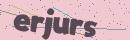# PHP采集运营类目微信商户类目

2020-06-11 18:09:27Laravel 的安装就不多说了, 不会安装可以看官方文档.

QueryList 的安装很简单, 可以直接看官网: www.querylist.cc

`composer require jaeger/querylist`

```public function start()
{
\$url = 'https://kf.qq.com/faq/140225MveaUz1501077rEfqI.html';
\$html = file_get_contents(\$url);
\$table = QueryList::html(\$html)->find('table');

// 如果需要表头 从 0 开始
\$table_rows = \$table->find('tr:gt(1)')->map(function(\$row) {
return \$row->find('td')->texts()->all();
});

\$rows_content = \$table_rows->all();
//dd(\$rows_content);

\$lst_content = [];
\$i = 0;
\$now_parent_id = 0;

foreach (\$rows_content as \$row_content) {
if (count(\$row_content) <= 2) continue;
\$i++;
if (count(\$row_content) == 7) {
// 当前是顶级分类和二级分类
\$now_parent_id = \$i;
\$lst_content[\$i] = [
'id' => \$i,
'name' => \$row_content,
'parent_id' => 0,
'level' => 1,
'describe' => str_replace("\n", '', \$row_content),
];

// 二级分类
\$i++;
\$lst_content[\$i] = [
'id' => \$i,
'name' => \$row_content,
'parent_id' => \$now_parent_id,
'level' => 2,
'describe' => \$row_content,
];
} else {
// 当前只有二级分类
\$lst_content[\$i] = [
'id' => \$i,
'name' => \$row_content,
'parent_id' => \$now_parent_id,
'level' => 2,
'describe' => \$row_content,
];
}
}

return response()->json(\$lst_content);
}```id   name   parent_id   level   describeSQL 格式的数据下载(博主导出的):https://pan.baidu.com/s/1del_w5s6jKfv7PeEEQ2nzg